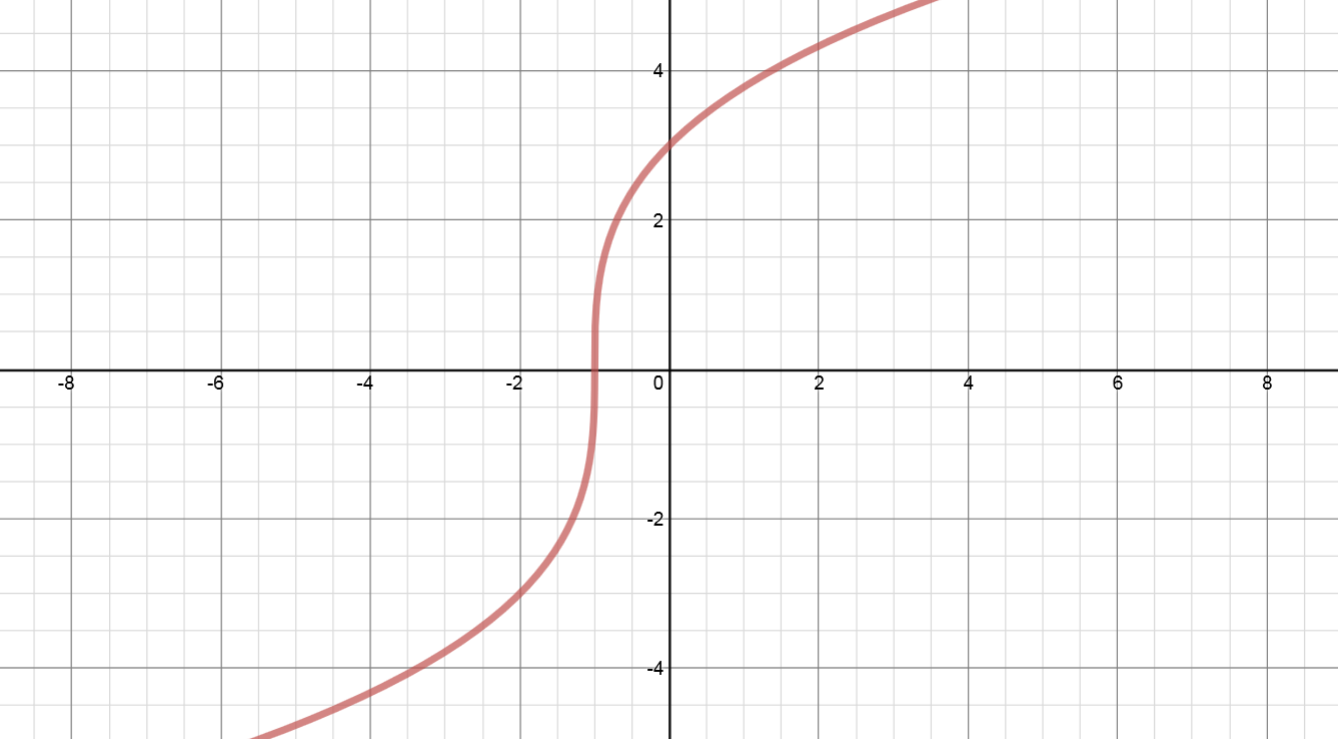# Transformations of functions: Horizontal translations#### Everything You Need in One Place

Homework problems? Exam preparation? Trying to grasp a concept or just brushing up the basics? Our extensive help & practice library have got you covered.#### Learn and Practice With Ease

Our proven video lessons ease you through problems quickly, and you get tonnes of friendly practice on questions that trip students up on tests and finals.#### Instant and Unlimited Help

Our personalized learning platform enables you to instantly find the exact walkthrough to your specific type of question. Activate unlimited help now!

0/2
##### Examples
###### Lessons
1. Sketch the following functions on the same set of coordinate axes:
$y = {\left( x \right)^2}$      VS.      $y = {\left( {x - 6} \right)^2}$      VS.      $y = {\left( {x + 5} \right)^2}$
2. Compared to the graph of $y = {x^2}$:
• the graph of $y = {\left( {x - 6} \right)^2}$ is translated "horizontally" ________ units to the ______________.
• the graph of $y = {\left( {x + 5} \right)^2}$ is translated "horizontally" ________ units to the ______________.
1. Horizontal Translations
Given the graph of $y = f\left( x \right)$ as shown, sketch:
1. $y = f\left( {x-8} \right)$
2. $y = f\left( {x+3} \right)$
3. In conclusion:
$\left( x \right) \to \left( {x-8} \right)$: shift __________ to the __________. All x coordinates $\Rightarrow$ ____________________
$\left( x \right) \to \left( {x+3} \right)$: shift __________ to the __________. All x coordinates $\Rightarrow$ ____________________###### Topic Notes
Horizontal translations refer to movements of a graph of a function horizontally along the x-axis by changing the x values. So, if y = f(x), then y = (x –h) results in a horizontal shift. If h > 0, then the graph shifts h units to the right; while If h < 0, then the graph shifts h units to the left.
Compared to $y=f(x)$:
$y=f(x-8)$: shift 8 units to the right
$y=f(x+3)$: shift 3 units to the left